ISSAC 94 - Symbolic-Numeric Nonlinear Equation Solving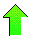Contents News View

 Application of the Mean-Value Theorem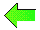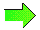From calculus we know that if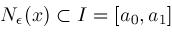then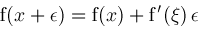for some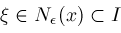.  If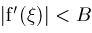for all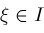and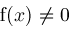, then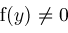for all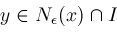where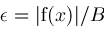.  Thus, while searching for zeros ofon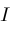, we can eliminate all of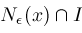from consideration if we knowand a finite bound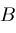.  If intervalis sufficiently small, then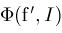will determine a finite boundon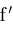.  This leads to procedure Narrow(,) which trims the ends of intervalby this technique before calling Zeros (if it must).  The recursive calls in Zeros are changed to be calls to procedure Narrow

We change lines 13 and 19-20 of procedure Zeros to be:return Narrow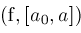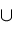Narrow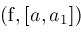;return Narrow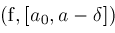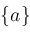Narrow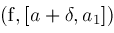;

and add procedure Narrow below:

proc Narrow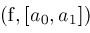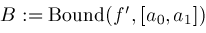;
if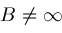thenforfrom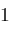to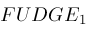do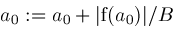;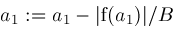;if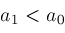thenreturn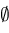;fi;od;
fi;
return Zeros;

proc Bound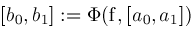;
return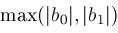;is a "fudge factor" which we currently set as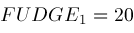©2004-2021 Planet Quantum Kelly Roach